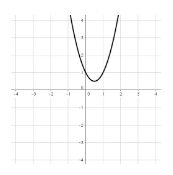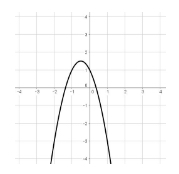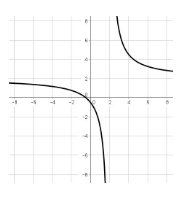• Turn off Ads!
•Create A Profile

# Page 2 Mathematics Study Guide for the TSI

### Intermediate Algebra and Functions

Intermediate algebra and functions includes more advanced operation with polynomials, working with exponents and rational expressions, and analyzing graphs of functions.

#### Polynomials

A monomial is a variable expression of the form $ax^{n}$.

$a$ represents a number and is called the coefficient.

The variable is raised to the power $n$.

A polynomial is an expression of different monomials added to, or subtracted from, each other.

The highest power of the variable is the degree of the polynomial.

Each monomial member of the polynomial is called a term.

If a function $f(x)$ is equal to a polynomial it is called a polynomial function.

Operations with Polynomials

Terms with the same variable(s) raised to the same power are called like terms. When adding or subtracting polynomials, only the like terms can be combined. The result of the operation will be a new, possibly simpler, polynomial.

When multiplying polynomials, all the terms in one polynomial must be multiplied against all the terms in the second one, and then like terms are combined. When both polynomials to be multiplied have 2 terms, there is a mnemonic called FOIL to remember the process: Multiply First terms, then Outer terms, then Inner terms, then Last terms.

Consider the following unsimplified polynomial:

To simplify, follow the order of operations:

which further simplifies to

Factoring Polynomials

Factoring a polynomial is the process of finding simpler polynomials that, when multiplied, will give the original polynomial as a result. Factoring may involve finding a common factor in each of the coefficients, and common factors of the variable(s). For example:

$3x^{2} + 6x^{3}$ can be factored to $3x^{2}(1 + 2x)$

Often, factoring involves re-writing polynomials to match known patterns.
One such pattern is:

A polynomial with one variable and degree 2 is called a quadratic, and functions equal to a quadratic are called quadratic functions.
Quadratic functions can be written in the form:

Parabola

The graph of a quadratic function is a parabola. Parabolas resemble the letter U and can open upward, if $a$ is positive or downward, if $a$ is negative:

Consider the following parabola with $a > 0$, $f(x) = 2x^2 - 2x + 1$, which graphs as:Now consider the following parabola with $% $, $f(x) = -2x^2 - 2x + 1$, which graphs as:Notice that the positive parabola has its vertex as its lowest point, and that the negative parabola has its vertex as its highest point.

Vertex

The vertex is the highest or lowest point of the parabola, where it changes direction.

Discriminant

The quantity $(b^{2} - 4ac)$ is called the discriminant of the quadratic function. If the discriminant is negative, the parabola does not cross the x-axis and there are no solutions (also called roots or zeroes) that are real numbers.
If the discriminant is zero, the vertex of the parabola touches the x-axis and there is one real solution.
If the discriminant is positive, the parabola crosses the x-axis in two places and there are two real solutions.

Quadratic equations are in the form $ax^{2} + bx +c = 0$. They can be solved by factoring or by using the quadratic formula. Factoring often involves using the pattern $(x + A)(x + B) = x^{2} + (A + B)x + AB$. If you can get the equation to the form $(x + A)(x + B) = 0$, then $(x + A)$ or $(x + B)$ (or both) must be zero, since the product is zero.

This is an example of a quadratic equation:

$f(x) = 3x^2 - x + 5$, with $a = 3$, $b = -1$, and $c = 5$.

#### Exponents and Roots

The expression $a^{n}$ indicates that the number a is raised to the nth power. Here, a is the base and n is the exponent. Positive exponents indicate how many times to multiply the base times itself. $a^{3}$ means $a\; \times\; a\; \times\; a$.

A root is the inverse operation of raising to a positive exponent. $\sqrt{a}$ means to find a number that, when raised to the third power, equals a.
A root is equivalent to raising to a fractional exponent: $\sqrt{a} = a^{\frac{1}{3}}$
$\sqrt{a}$, with no root number shown, means the square root, a root number of 2.

There are several rules for working with exponents:

#### Order of Operations

When an expression has a variety of operations, you must follow the order of operations to get the correct result. The order of operations is this:

Do everything in parentheses (P), left to right.
Evaluate any exponents (E), left to right.
Do all multiplication and division (MD), in order, left to right.
Then do all addition and subtraction (AS), in order, from left to right.

Good way to remember: Please Excuse My Dear Aunt Sally

#### Rational Equations

Rational equations have a fraction with a polynomial expression in both numerator and denominator set equal to a constant. Often, it is easier to solve them by factoring both polynomials in the hope that there is a common factor that can be canceled. Otherwise, they are solved as for any other equation by performing identical operations to both sides and simplifying until the solution is found.

This is an example of a rational equation:

$\frac{3x - 1}{4} = \frac{x + 3}{9}$, which is solved by cross multiplying and isolating x:

$9(3x - 1) = 4(x + 3)$, which becomes

$27x - 9 = 4x + 12$, which becomes

$23x = 21$, or $x = \frac{21}{23}$.

#### Rational Functions

Rational functions are similar to rational equations, except that the fraction is set equal to a second variable. Since there is a denominator, there exists the possibility that the denominator is zero for some value of x. In that case, the function is not defined for that value of x and there is a break in the graph at that point. For example, the function $y = \frac{2x + 1}{x-2}$ is undefined at $x = 2$, because that value of $x$ results in a denominator of zero. Its graph would look like this:Notice that the function gradually approaches $x = 2$, but never touches it; $x = 2$ is a vertical asymptote.